# GASES BEHAVIOR LAWS AND KINETIC MOLECULAR THEORY Kenneth

• Slides: 33
Download presentationGASES BEHAVIOR, LAWS, AND KINETIC MOLECULAR THEORY Kenneth E. Schnobrich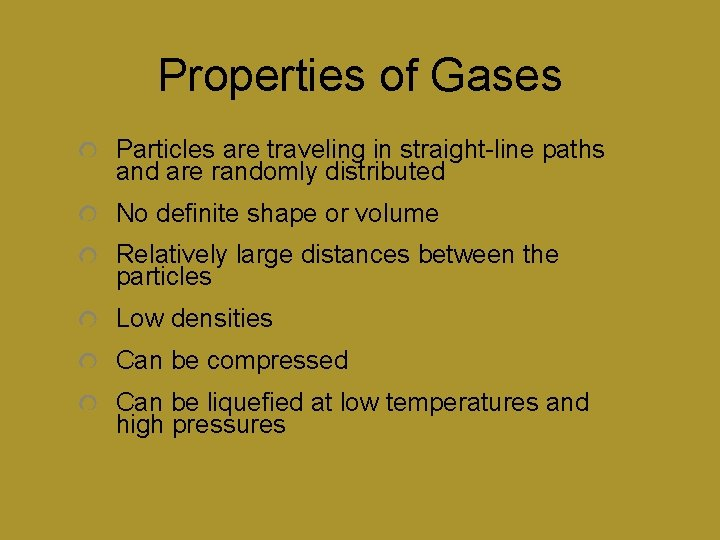Properties of Gases Particles are traveling in straight-line paths and are randomly distributed No definite shape or volume Relatively large distances between the particles Low densities Can be compressed Can be liquefied at low temperatures and high pressures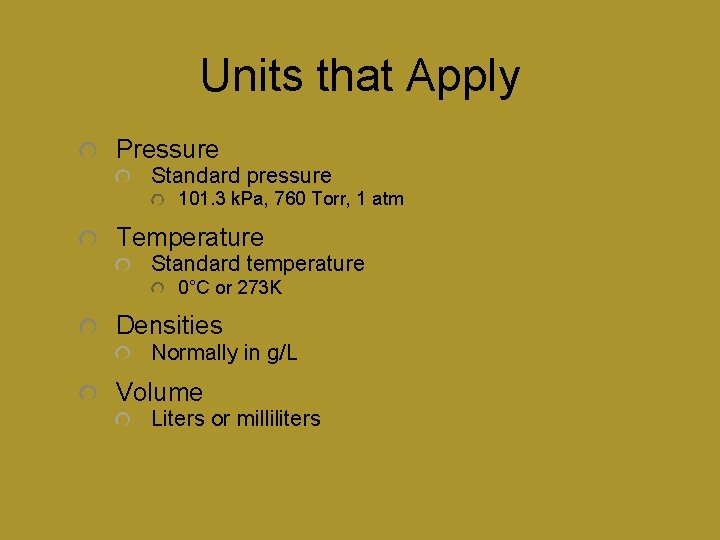Units that Apply Pressure Standard pressure 101. 3 k. Pa, 760 Torr, 1 atm Temperature Standard temperature 0°C or 273 K Densities Normally in g/L Volume Liters or millilitersGas Behavior and the Gas Laws Boyle’s Law This law examines the relationship between volume and pressure The volume occupied by a gas is inversely proportional to the pressure exerted on the gas V a 1/PSample Problem Always identify the variables - P 1, P 2, V 1, and V 2. Use the relationship on “Table T” (P 1 V 1 = P 2 V 2) - note temperature is constant. What volume will 500. m. L of a gas occupy if the pressure is changed from 101. 3 k. Pa to 502. 4 k. Pa? What do you definitely know? ?Solution You definitely know that the volume should decrease because pressure is increasing P 1 V 1 = P 2 V 2 (101. 3 k. Pa)(500 m. L) = (502. 4 k. Pa)(V 2) V 2 = 500. m. L(101. 3 k. Pa/502. 4 k. Pa) V 2 = 100. 8 m. L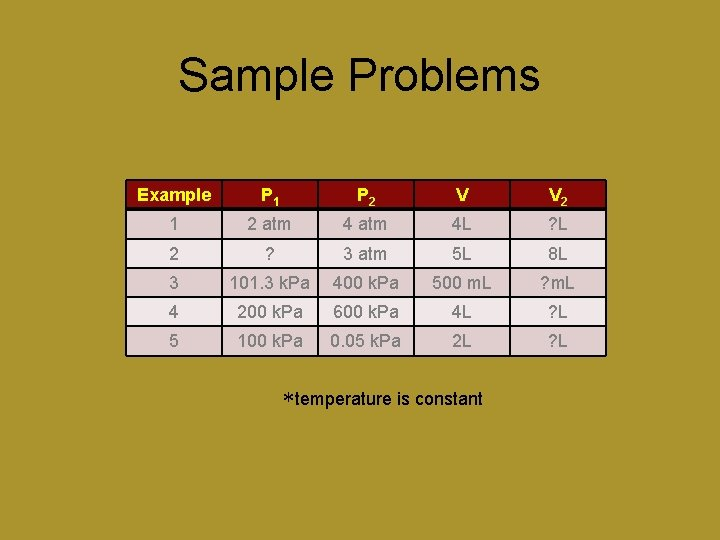Sample Problems Example P 1 P 2 V V 2 1 2 atm 4 L ? L 2 ? 3 atm 5 L 8 L 3 101. 3 k. Pa 400 k. Pa 500 m. L ? m. L 4 200 k. Pa 600 k. Pa 4 L ? L 5 100 k. Pa 0. 05 k. Pa 2 L ? L *temperature is constant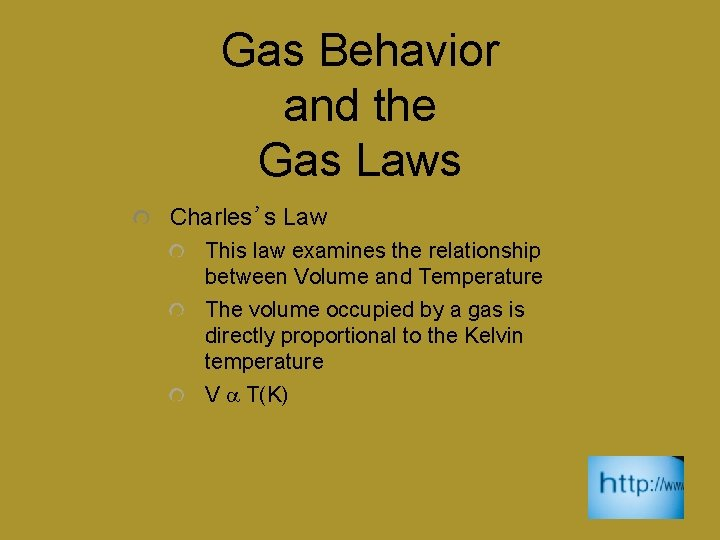Gas Behavior and the Gas Laws Charles’s Law This law examines the relationship between Volume and Temperature The volume occupied by a gas is directly proportional to the Kelvin temperature V a T(K)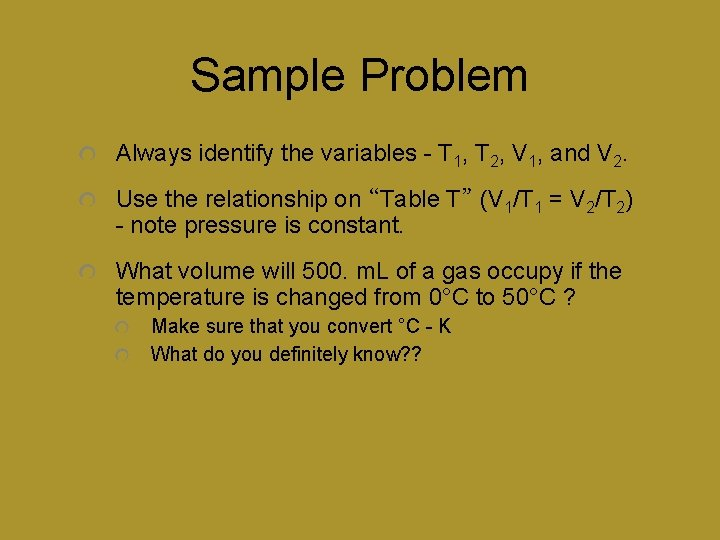Sample Problem Always identify the variables - T 1, T 2, V 1, and V 2. Use the relationship on “Table T” (V 1/T 1 = V 2/T 2) - note pressure is constant. What volume will 500. m. L of a gas occupy if the temperature is changed from 0°C to 50°C ? Make sure that you convert °C - K What do you definitely know? ?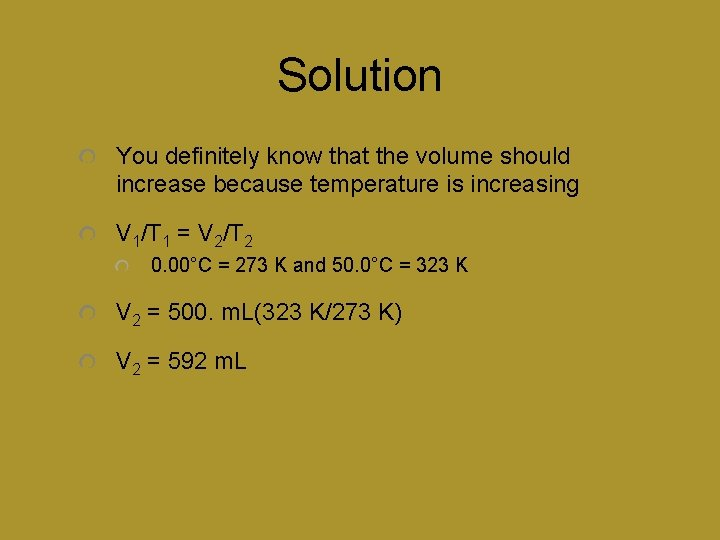Solution You definitely know that the volume should increase because temperature is increasing V 1/T 1 = V 2/T 2 0. 00°C = 273 K and 50. 0°C = 323 K V 2 = 500. m. L(323 K/273 K) V 2 = 592 m. LSample Problems Example V 1 V 2 T 1 T 2 1 400 m. L ? m. L 25°C 50°C 2 4 L 10 L ? K 819 K 3 8 L 10 L 273 K ? K 4 ? L 20 L 819 K 273 K 5 10 L 3 L 10°C ? K *Pressure is constantThe Combined Gas Law The combined gas law simply deals with both change in pressure and change in temperature (Table T)1. P 1 V 1/T 1 = P 2 V 2/T 2 In this problem, as we have with Charles’s and Boyle’s Laws, the number of mols of gas remains constant. 1 New York State Reference Tables for ChemistrySample Problem 20. L of H 2 gas is at a pressure of 101. 3 k. Pa and 20°C. What volume will this gas occupy at a pressure of 20. 6 k. Pa and a temperature of 0°C? Start by identifying your variables in the problem: P 1, V 1, T 1, P 2, T 2 - solve for V 2 # mols of gas is constant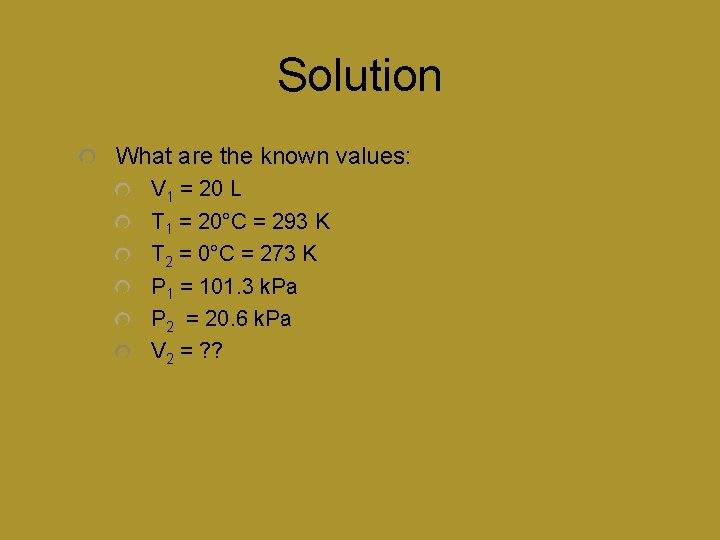Solution What are the known values: V 1 = 20 L T 1 = 20°C = 293 K T 2 = 0°C = 273 K P 1 = 101. 3 k. Pa P 2 = 20. 6 k. Pa V 2 = ? ?Solution (cont. ) V 2 = V 1(T 2/T 1)(P 1/P 2) Notice ü Since the temperature is decreasing it will decrease the value for V 1 (direct relationship) Since the pressure is decreasing it will increase the value for V 1 (inverse relationship) V 2 = 20. L(273 K/293 K)(101. 3 k. Pa/20. 6 k. Pa) V 2 = 92 L (to the correct # of significant figures)Sample Problems Trial V 1 P 1 T 1 V 2 P 2 T 2 1 10 L 2 atm 373 K ? L 4 atm 456 K 2 5 L ? atm 200 K 10 L 2 atm 400 K 3 2 L 4 atm 20°C ? L 0. 5 atm 373 K **mols of gas constant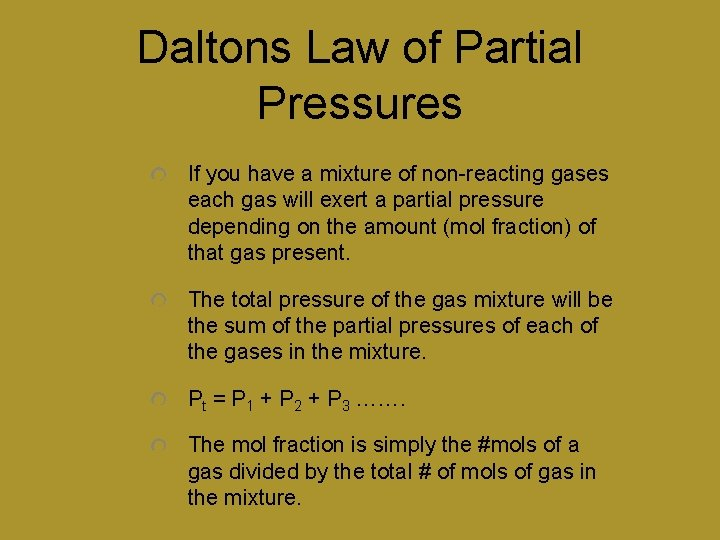Daltons Law of Partial Pressures If you have a mixture of non-reacting gases each gas will exert a partial pressure depending on the amount (mol fraction) of that gas present. The total pressure of the gas mixture will be the sum of the partial pressures of each of the gases in the mixture. Pt = P 1 + P 2 + P 3 ……. The mol fraction is simply the #mols of a gas divided by the total # of mols of gas in the mixture.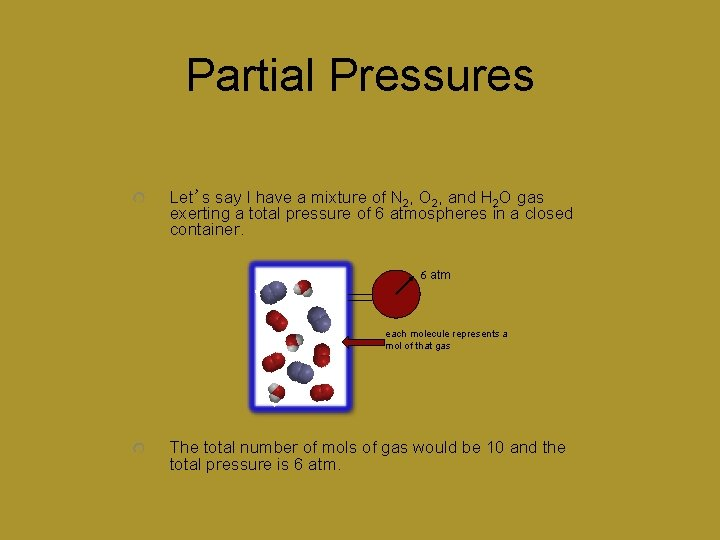Partial Pressures Let’s say I have a mixture of N 2, O 2, and H 2 O gas exerting a total pressure of 6 atmospheres in a closed container. 6 atm each molecule represents a mol of that gas The total number of mols of gas would be 10 and the total pressure is 6 atm.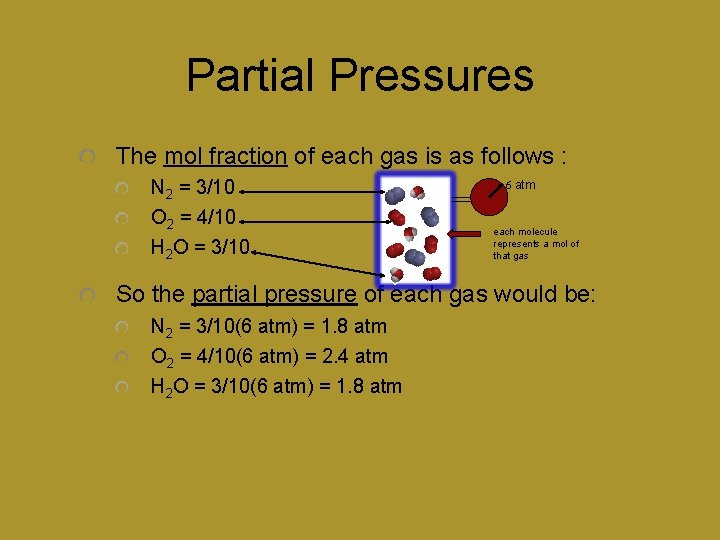Partial Pressures The mol fraction of each gas is as follows : N 2 = 3/10 O 2 = 4/10 H 2 O = 3/10 6 atm each molecule represents a mol of that gas So the partial pressure of each gas would be: N 2 = 3/10(6 atm) = 1. 8 atm O 2 = 4/10(6 atm) = 2. 4 atm H 2 O = 3/10(6 atm) = 1. 8 atm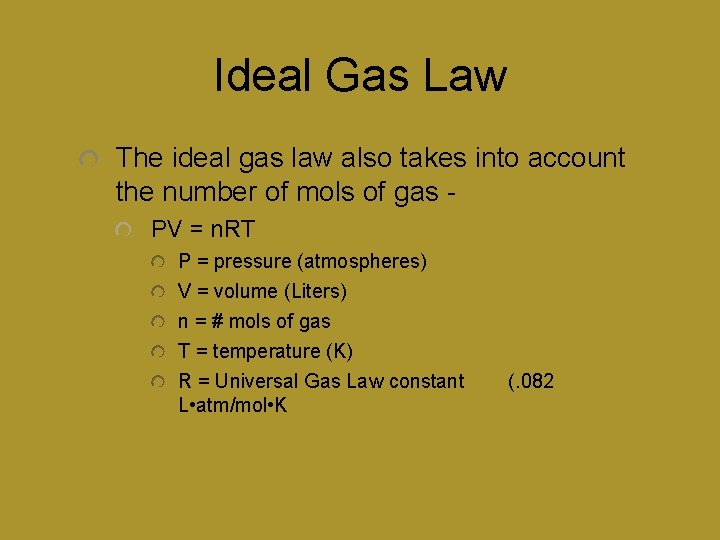Ideal Gas Law The ideal gas law also takes into account the number of mols of gas PV = n. RT P = pressure (atmospheres) V = volume (Liters) n = # mols of gas T = temperature (K) R = Universal Gas Law constant L • atm/mol • K (. 082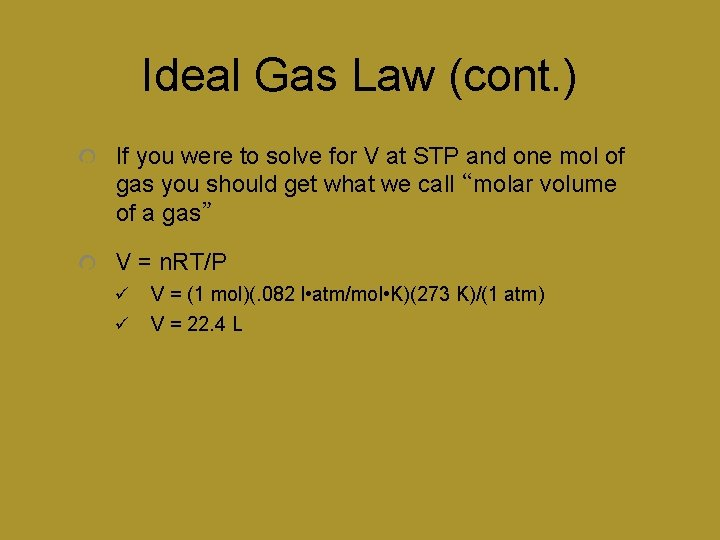Ideal Gas Law (cont. ) If you were to solve for V at STP and one mol of gas you should get what we call “molar volume of a gas” V = n. RT/P ü ü V = (1 mol)(. 082 l • atm/mol • K)(273 K)/(1 atm) V = 22. 4 LMolar Volume Experimentally it can be determined that one mol of any gas, at STP, will occupy a volume of 22. 4 L STP = standard temperature & pressure = 0°C(273 K) and 1 atm(101. 3 k. Pa)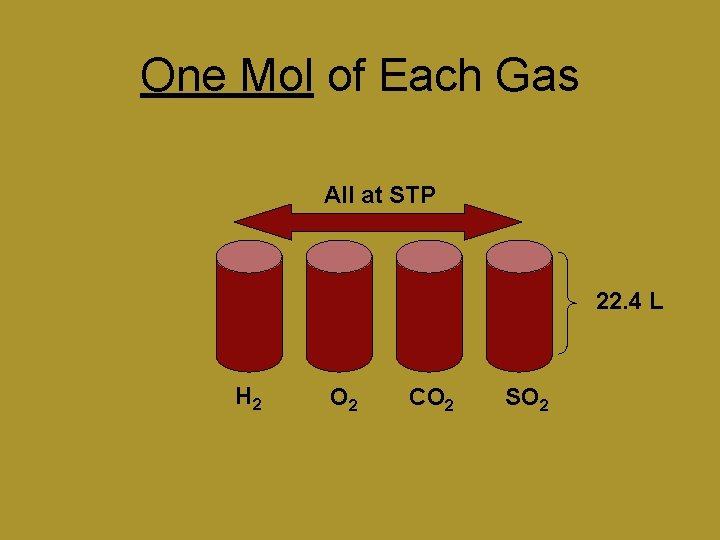One Mol of Each Gas All at STP 22. 4 L H 2 O 2 CO 2 SO 2Avogadro’s Hypothesis Equal volumes of gases under the same conditions of temperature and pressure will contain equal #’s of particles 1. 0 L Same Volume Same Temperature & Pressure EQUAL NUMBER OF PARTICLES 1. 0 L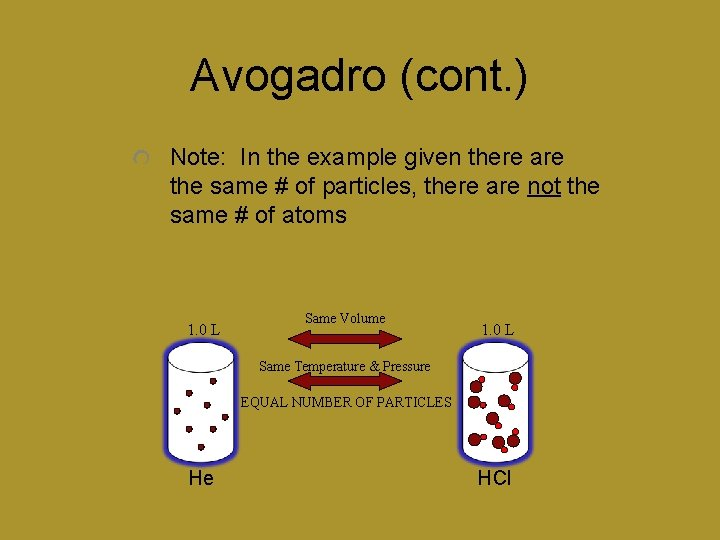Avogadro (cont. ) Note: In the example given there are the same # of particles, there are not the same # of atoms 1. 0 L Same Volume 1. 0 L Same Temperature & Pressure EQUAL NUMBER OF PARTICLES He HClGraham’s Law of Diffusion - the movement of particles from a region of high concentration to a region of low concentration Effusion - the movement of particles from a region of high concentration to a region of low concentration through a small orifice (small opening)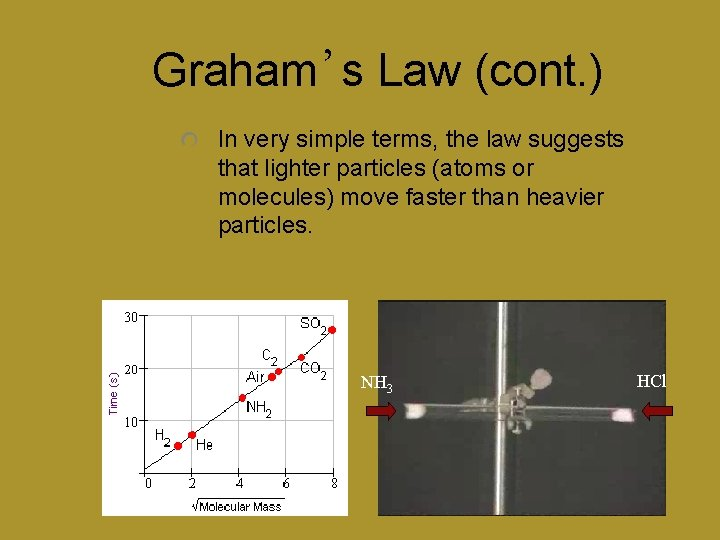Graham’s Law (cont. ) In very simple terms, the law suggests that lighter particles (atoms or molecules) move faster than heavier particles. NH 3 HCl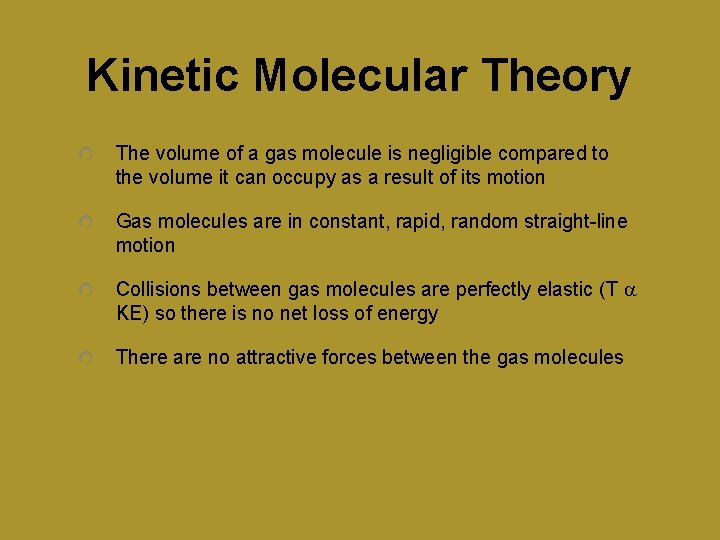Kinetic Molecular Theory The volume of a gas molecule is negligible compared to the volume it can occupy as a result of its motion Gas molecules are in constant, rapid, random straight-line motion Collisions between gas molecules are perfectly elastic (T a KE) so there is no net loss of energy There are no attractive forces between the gas molecules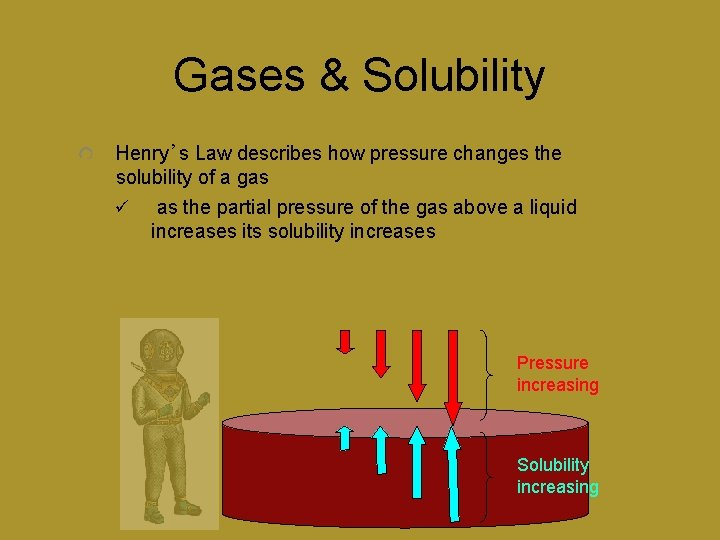Gases & Solubility Henry’s Law describes how pressure changes the solubility of a gas ü as the partial pressure of the gas above a liquid increases its solubility increases Pressure increasing Solubility increasingGases & Solubility As temperature increases the solubility of a gas will decrease ü when gases normally go into solution it is an exothermic process and for that reason when the temperature of solutions of gases in liquids increases the solubility decreases ü Like your soda going flat when you leave it out on a warm day - the increased temperature causes the CO 2 to come out of solution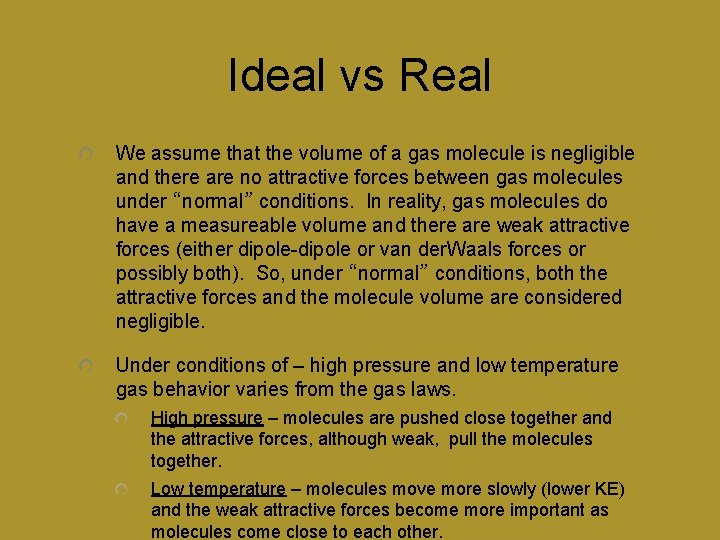Ideal vs Real We assume that the volume of a gas molecule is negligible and there are no attractive forces between gas molecules under “normal” conditions. In reality, gas molecules do have a measureable volume and there are weak attractive forces (either dipole-dipole or van der. Waals forces or possibly both). So, under “normal” conditions, both the attractive forces and the molecule volume are considered negligible. Under conditions of – high pressure and low temperature gas behavior varies from the gas laws. High pressure – molecules are pushed close together and the attractive forces, although weak, pull the molecules together. Low temperature – molecules move more slowly (lower KE) and the weak attractive forces become more important as molecules come close to each other.Ideal vs Real So, gases behave most ideally at high temperatures (molecules are moving too fast for the weak attractive forces to have an effect) and low pressures/large volumes (molecules are so far apart that the attractive forces and molecule volume are insignificant). There are only two gases that behave close to ideally under all conditions and they are – H 2 and He. They both have very small molecule volumes and extremely weak van der Waals forces. H 2 HeIdeal vs Real Critical temperature – above this temperature the substance cannot exist as a liquid. Critical pressure – at the critical temperature, the pressure that must be applied to cause that gas to condense H 2 He -240°C -268°C Critical Temperatures (°C)